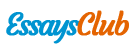# Ols to Estimate a Model Relating Median Housing Price in the Community to Various Community Characteristics

Autor:   •  April 29, 2018  •  Research Paper  •  2,852 Words (12 Pages)  •  297 Views

Page 1 of 12

## Question 1

1. ‘Use OLS to estimate a model relating median housing price in the community to various community characteristics given by the equation below and present the estimated equation, including the standard errors of estimated coefficients and R2.’

[pic 1]

In order to attain the estimated coefficients, their standard errors and R2, the data set, containing 506 cross-sectional observations induvial sets of data, was entered into Eviews. Eviews then estimated an equation mimicking the one above using the data set and following results were observed [pic 2]

The equation was then developed using the coefficient for each variables and their standard errors, found under the ‘coefficient’ and ‘Std. Error’ columns respectively. The R2 was found in the top left hand corner in the bottom half of the table. These results are shown below.

[pic 3]

[pic 4]

The standard error for each variable can be found in the table below.

 Variable Standard Error C 0.282 crime 0.001 dist 0.007 ln(nox) 0.92 lowstat 0.002 ln(proptax) 0.048 radial 0.002 stratio 0.005 rooms 0.016

1. ‘At the time the data was collected, a real estate agent claimed that an extra room added 15% to the price of a house. Test that null hypothesis against the alternative hypothesis that it adds less than 15%. (5 marks)’

In order to test this claim the null hypothesis test will be used at a 5% significance level. Now this test refers to the coefficient 𝐵9 and its effect on the price of a house. If this this claim was true that would mean that 𝐵9 =0.15. The alternative hypothesis for this claim is that an extra room adds less than 15%, meaning 𝐵9 < 0.15. This hypothesis is tested below.

Hypothesis         and [pic 5][pic 6]

Decision Rule         Reject  if [pic 7][pic 8]

Test Statistic         [pic 9]

Decision         Reject [pic 10]

Conclusion         An extra room inside an house will increase the price by less that 15% in GDP due to an 1% increase of the labor force in the agricultural sector is different from 0, at a 5% significance level, after controlling for other variables at a 5% significance level

1. ‘Plot the residuals of your regression against dist and comment. (2 marks)’

Below is a scatter plot highlighting the residuals of the regression against dist. [pic 11]

As you can see, the graph plots the variation of the residuals of the estimated equation. It can be observed that as the value of dist. increases, this variation becomes smaller and smaller. When dist. was 2, the residuals spanned between -0.8 and 0.8 however when dist. was 12, the residuals were between 0 and 0.2. This is strong evidence of heteroscedasticity.

1. ‘Use White’s test (without cross products) to test for heteroskedastic errors. What do you conclude? (5 marks)’

In order to test for heteroskedastic errors, Eviews was used to run a White heteroskedastic test. This table, shown below, was given as the output.

[pic 12]

The R-squared value was then used to run a White test. This test can be seen below

Hypothesis         and [pic 13][pic 14]

Decision Rule         Reject  if )[pic 15][pic 16]

Test Statistic         [pic 17]

Decision         Reject [pic 18]

Conclusion         The is evidence of heteroscedasticity at a 5% significance level

1. ‘Conduct a Goldfeld-Quandt test of the null hypothesis of no heteroscedasticity against the alternative that the variances of the errors are changing with dist. Explain how you choose two groups of the data to perform the test. (6 marks)’

In order to conduct a Goldfeld-Quandt test, the data set must be split into two groups. After analyzing the variation of the residuals in the scatter plot in question c) above, it was decided that the data would be split where dist. equaled 3. This is because the variation was larger when dist was larger than 3 when compared the variation when dist was smaller than 3. The two data sets where then entered into Eviews and the following output was received. [pic 19][pic 20]

^ Eviews output when dist < 3                                                ^ Eviews output when dist > 3

The standard error of both regressions ( was then used to run a Goldfeld-Quandt test. This test can be seen below[pic 21]

Hypothesis        and [pic 22][pic 23]

Decision Rule         Reject  if [pic 24][pic 25]

Test Statistic         [pic 26]

Decision         Reject [pic 27]

Conclusion         The error variance of the first group is statistically larger than that of the second group meaning there is evidence of heteroscedasticity, at a 5% significance level

1. ‘Perform a Breusch-Pagan test of the null hypothesis of no heteroscedasticity against the alternative hypothesis that. (5 marks)’[pic 28]

In order to test for heteroskedastic errors, Eviews was used to run a Breusch-Pagan-Godfrey heteroskedastic test. This table, shown below, was given as the output.

[pic 29]

The R-squared value was then used to run a Breusch-Pagan test. This test can be seen below

Hypothesis         and  [pic 30][pic 31]

Decision Rule         Reject  if [pic 32][pic 33]

Test Statistic         [pic 34]

Decision         Reject [pic 35]

Conclusion         There is evidence of heteroscedasticity in the form of., at a 5% significance level[pic 36]

...